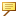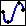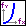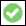> > Create a 2D functional curve

Create a 2D functional curve
Creo Elements/Direct Modeling represents 2D curves as straight lines, circles, and freeform (b-spline) curves. Normally, you draw curves and circles directly on the workplane. If you use the Function command, you can create a curve based on a mathematical calculation containing functional expressions such as polynomials, trigonometric functions, or exponential functions.
The resulting curve cannot be represented exactly, so Creo Elements/Direct Modeling generates a b-spline curve that approximates the expression. You can specify a tolerance to define the maximum distance allowed between the exact curve and the representation that is generated.
For example, you can draw the following sinus curve by entering mathematical functions. You can follow the link to other examples at the bottom of this page.The calculation is done in millimeters (mm) and not in the units you have set in your system settings. Creo Elements/Direct Modeling cannot convert user-defined units when it approximates the curve.
To create an approximate functional curve,
1. Click Modeling and then, in the Draw group, click the arrow next toSpline.
2. ClickFunction in the Functional section. The Function dialog box opens.
3. Change the Variable, if necessary. Any combination of normal Roman letters is allowed. Special characters, such as ü or @ are not allowed. You should use this variable in your Function, Start, and End expressions.
4. Type your Function. See Using mathematical expressions for a list of valid expressions and operators.
5. Type Start and End values. These determine the interval on which the curve is defined, or the range of all values the variable will calculate.
6. Type a Tolerance, which determines how far the represented curve can be from the exact curve.
7. Click OKto complete the operation.
Limitations
Creo Elements/Direct Modeling cannot calculate logarithmic functions in your expressions.